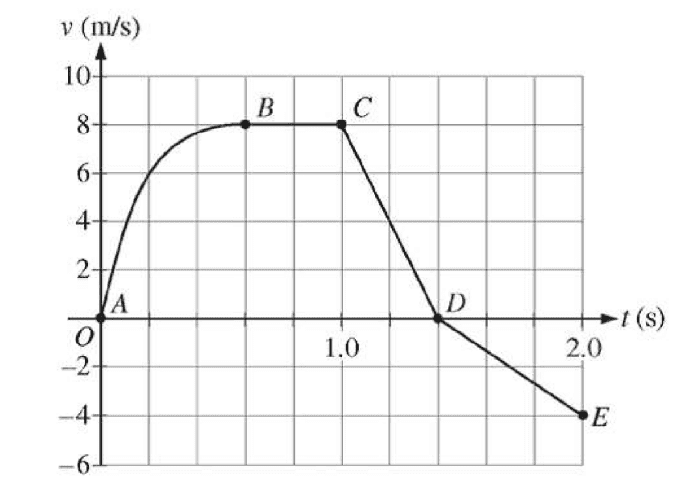# Energy with varying force

• Altoid
However, this logic is not correct.In summary, the logic behind determining work done by acceleration is flawed.f

#### Altoid

Homework Statement
A cart is constrained to move along a straight line. A
varying net force along the direction of motion is exerted on
the cart. The cart’s velocity v as a function of time tis
shown in the graph above. The five labeled points divide
the graph into four sections.
During some part of the motion, the work done on the cart
is negative. What feature of the motion indicates this?
A. Speed is increasing
B. Speed is decreasing
C. Acceleration is increasing
D. Acceleration is decreasing (INCCORECT)
Relevant Equations
W = (f)(d)(cos(theta))
Knowing that negative work occurs when the force applied to an object opposes the direction of displacement, and that the direction of acceleration vector should align with the force vector, I assumed the correct answer was that the indication of negative work comes from negative acceleration, however this is incorrect, does anyone know why this logic is faulty/doesn't work?
This is the graph btw:Consider the segment between B and C. The velocity of the cart is constant, so acceleration is zero. No work is being performed there. Next consider the segment between C and D. What happens there? Is acceleration increasing, decreasing, or constant?

Consider the segment between B and C. The velocity of the cart is constant, so acceleration is zero. No work is being performed there. Next consider the segment between C and D. What happens there? Is acceleration increasing, decreasing, or constant?
Acceleration is constant, and negative

So, by your own words, "Acceleration is decreasing" is not correct, yes?

So, by your own words, "Acceleration is decreasing" is not correct, yes?
OHHHH, Acceleration is not decreasing, speed is decreasing because their is a negative acceleration.

Yes!

Yes!
Thanks!

•gneill
Acceleration is constant, and negative
OHHHH, Acceleration is not decreasing, speed is decreasing because their is a negative acceleration.
You have to be careful with "negative" acceleration. Acceleration is a vector and can be positive or negative depending on how you define your coordinate axes. The same is true about velocity. So "positive" and "negative" don't mean much because speed and work are scalar quantities that do not depend on the choice of coordinate axes. Here is a summary of how to look at it.

In one dimension, once you have defined the axes,
1. If the velocity and the acceleration are in the same direction (positive or negative) the speed is increasing and the work done on the moving object is positive.
2. If the velocity and the acceleration are in opposite directions (one positive and one negative) the speed is decreasing and the work done on the moving object is negative.
3. If the acceleration is zero, the direction of the velocity does not matter, the speed stays constant and the work done on the moving object is zero.
With this in mind, it should be easy to look at any velocity vs. time graph and figure out over what interval the external force does positive and negative work.